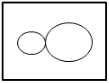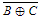## UGC NET CS 2010 June-Paper-2

 Question 1
“x1 is a clone of x” means x1 is identical to x in terms of the physical attributes namely, height, weight and complexion. Given, height, weight and complexion only form a complete set of attributes for an entity, cloning is an equivalence relation. What is your impression about this statement ?
 A The statement is true B The statement is false C The truth value of the statement cannot be computed D None of these
Engineering-Mathematics       Set-Theory
Question 1 Explanation:
The given statement says that the Relation R contains x and x1 which are identical. Which means (x,x1) are same as (x,x).
We know that " a relation of (x,x) type is an equivalence as it satisfies Reflexive, symmetric, transitive.
The answer would be option A, it is equivalence.
 Question 2
‘R is a robot of M’ means R can perform some of the tasks that otherwise M would do and R is unable to do anything else. Which of the following is the most appropriate representation to model this situation ?
 ABCD None of these
Engineering-Mathematics       Set-Theory
Question 2 Explanation:
Given data,
-- R is a robot of M
Step-1: Constraint is the R can perform some tasks of M. It means M can do all tasks.
But sometimes R can’t do which can do by M.
Step-2: The above constraint clearly representing that M is superset and R is subset of M.
We can represent this in venn diagrams areQuestion 3
“My Lafter Machin(MLM) recognizes the following strings :
(i) a
(ii) aba
(iii) abaabaaba
(iv) abaabaabaabaabaabaabaabaaba
Using this as an information, how would you compare the following regular expressions ?
(i) (aba)3x
(ii) a.(baa)3x–1. ba
(iii) ab.(aab).3x–1.a
 A (ii) and (iii) are same, (i) is different. B (ii) and (iii) are not same. C (i), (ii) and (iii) are different. D (i), (ii) and (iii) are same.
Theory-of-Computation       Regular-Expression
Question 3 Explanation:
m is generating single "a".
If you observe all of them is generating (aba)x
Note: This question is ambiguous, the wordings are not very clear.
 Question 4
S1 : I teach algorithms and maths.
S2 : My professor teaches maths, electronics and computer science.
S3 : I have a student of maths.
S4 : Algorithm is a part of computer science.
S5 : Maths students know computer science.
What would be the chromatic number of a graph, vertices of which are the actors/entities that are involved in the sentences S1 to S5 and edges-to represent the associations/relationships amongst the entities/actors as expressed in the sentences S1 to S5 above ?
 A 2 B 3 C 4 D None of these
Engineering-Mathematics       Graph-Theory
 Question 5
Four your ATM debit card, you have a 4-decimal-digit personal secret code. In the absence of any clue, a brute-force attack takes time-‘t’ to crack the code on an ATM terminal. Therefore ‘t’ is the secure-time for a customer to report in case the card is misplaced. Your Bank has decided to facilitate an increased secure-time. Out of the following, which option should provide the largest rise in the value of ‘t’ ?
 A Instead of 4-decimal-digits, maintain the personal secret code in 4-hexadecimal-digits. B Instead of 4-decimal digits, maintain a 5-decimal-digit personal secret code. C Reduce the processing speed of the ATM terminals to the half of their current speed. D None of the above provides any improvement.
Computer-Networks       Security
Question 5 Explanation:
→ To get the password of 4 digits possible number digit would be 104 which has brute-force attack takes time-‘t’.
Therefore ‘t’ is the secure-time for a customer to Increase the secure more time we have to increase the size of the password of 5 digits or more.
 Question 6
The logic expression for the output of the circuit shown in the figure isA A’C’ + B’C’ + CD B AC’ + BC’ +C’D C ABC + C’D’ D A’B’ + B’C’ + C’D’ E None of the above
Digital-Logic-Design       Logic-Gates
Question 6 Explanation:
[((A+B)'+c)'+(c+d)']'
=((A+B)'+C)(C+D)
=(A'B'+C)(C+D)
=A'B'C+C+A'B'D+CD
=(A'B'+1+D)+A'B'D
=A'B'D
Note: They given wrong options.
 Question 7
Advantage of synchronous sequential circuits over asynchronous ones is
 A faster operation B ease of avoiding problems due to hazard C lower hardware requirement D better noise immunity E None of the above
Digital-Logic-Design       Sequential-Circuits
Question 7 Explanation:Note: Excluded for evaluation.
 Question 8
What is the transitive voltage for the voltage input of a CMOS operating from 10V supply ?
 A 1V B 2V C 5V D 10 V
Digital-Logic-Design       Logic-Gates
 Question 9
What is decimal equivalent of BCD 11011.1100 ?
 A 22.0 B 22.2 C 20.2 D 21.2 E None of the above
Digital-Logic-Design       Number-Systems
Question 9 Explanation:
The question is not properly framed.
In the question it is mentioned that 11011.1100 is BCD.
We know that BCD code is different from Binary number system.
Each of the decimal digit has a 4-bit binary code or in other words every block of 4 bits has a corresponding decimal digit.
0000- 0
0001- 1
:
:
1001- 9
1010 - 1111 are not valid codes.
If the given code 11011.1100 is BCD then divide it into blocks of 4 bits.
0001 1011. 1100 (Note: Appending zeros on the left side will not change value.)
But 1011 is not a valid BCD code.
 Question 10
The function represented by the k- map given below isA A ⋅ B B AB + BC + CA CD A . B . C
Digital-Logic-Design       K-Map
Question 10 Explanation:Note: Above options are wrong.
 Question 11
The statement
printf(“%d”,10 ? 0 ? 5 : 1 : 12);
will print
 A 10 B 0 C 12 D 1
Programming       Operator
Question 11 Explanation:
We can write above statement into (10 ? (0 ? 5 : 1) : 12)
Step-1: (0 ? 5 : 1) → It will give result 1 because the condition is FALSE because given value is 0. If nonzero given in condition, it will print TRUE value.
Step-2: (10 ? 1 : 12) → Here, given condition is nonzero. So, it will print TRUE value. The TRUE value is 1.
Note: Ternary operators(?:) evaluation starts from innermost conditions first.
 Question 12
What will be the output of the following c-code ?
void main ( )
{
char *P = "ayqm" ;
char c;
c = ++*p ;
printf ("%c", c);
}
 A a B c C b D q
Programming       Operator
Question 12 Explanation:
Pointer "p" will point to the string "ayqm",
*p means the first character of the string which is "a"
++*p means an increment of "a" which is nothing but "b"
printf ("%c", c); means the character "b" will be printed as output.
Note: They given syntax error in program.
There are 12 questions to complete.

Register Now